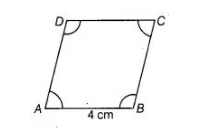# Opposite angles of a quadrilateral`
Question:

Opposite angles of a quadrilateral ABCD are equal. If AB = 4 cm, determine CD.

Solution:

Given, opposite angles of a quadrilateral are equal. So, ABCD is a parallelogram and we know that, in a parallelogram opposite sides are also equal.

∴ CD = AB = 4cm